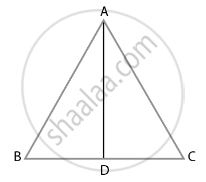# Find the altitude of an equilateral triangle of side 8 cm. - Mathematics

Diagram
Sum

Find the altitude of an equilateral triangle of side 8 cm.

#### Solution

Let ABC be an equilateral triangle of side 8 cm

AB = BC = CA = 8 cm. ....(All sides of an equilateral triangle is equal)Draw altitude AD which is perpendicular to BC.

Then, D is the mid-point of BC.

∴ BD = CD = 1/2

BC = 8/2 = 4 cm

Now, by Pythagoras theorem

⇒ (8)2 = AD2 + (4)2

⇒ 64 = AD2 + 16

⇒ AD = sqrt(48) = 4sqrt(3) cm.

Hence, altitude of an equilateral triangle is 4sqrt(3) cm.

Concept: Application of Pythagoras Theorem in Acute Angle and Obtuse Angle
Is there an error in this question or solution?

#### APPEARS IN

NCERT Mathematics Exemplar Class 10
Chapter 6 Triangles
Exercise 6.3 | Q 6 | Page 68

Share